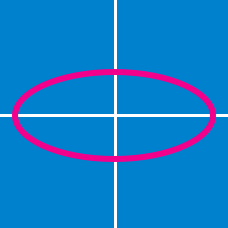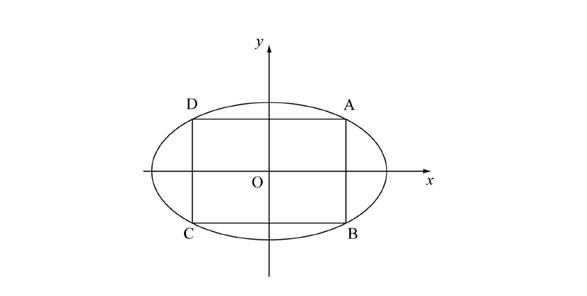Geometry

# Conics - Ellipse - General

Let $$(m,n)$$ be the point at which a line is tangent to the ellipse $$\displaystyle{\frac{x^2}{16}+\frac{y^2}{100}=1}.$$ If this line has $$x$$-intercept $$A=(a,0)$$ and $$y$$-intercept $$B=(0,b),$$ where $$a$$ and $$b$$ are positive, what is the minimum value of $$ab?$$

The ellipse $$\displaystyle{\frac{x^2}{8}+\frac{y^2}{17}=1}$$ and the line $$y=2x - k$$ intersect at exactly two distinct points. What is the range of the constant $$k?$$

Find the equation of the tangent line to the ellipse $$1x^2+y^2=53$$ at the point $$(2,7)$$ on the ellipse.In the above diagram, a rectangle $$ABCD$$ is inscribed in the ellipse $$\displaystyle{\frac{x^2}{36}+\frac{y^2}{100}=1}.$$ What is the maximum area of rectangle $$ABCD?$$

Note: The above diagram is not drawn to scale.

Find the maximum area of the rectangle which is inscribed in the ellipse $\frac{x^{2}}{5^{2}}+\frac{y^{2}}{2^{2}} = 1.$

×

Problem Loading...

Note Loading...

Set Loading...